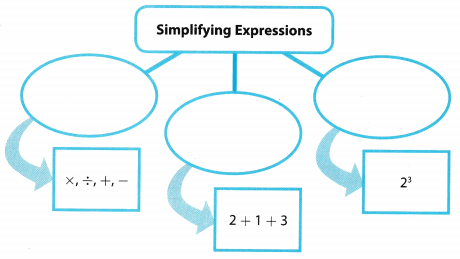# Texas Go Math Grade 6 Module 11 Answer Key Generating Equivalent Algebraic Expressions

Refer to our Texas Go Math Grade 6 Answer Key Pdf to score good marks in the exams. Test yourself by practicing the problems from Texas Go Math Grade 6 Module 11 Answer Key Generating Equivalent Algebraic Expressions.

## Texas Go Math Grade 6 Module 11 Answer Key Generating Equivalent Algebraic Expressions

Essential Question
How can you generate equivalent algebraic expressions and use them to solve real-world problems?

Complete these exercises to review skills you will need for this chapter.

Evaluate.

Question 1.
11 + (20 – 13)
Solution to this example is given below
11 + (20 – 13)
11 + (20 – 13) = 11 + 7 Perform operations inside parentheses. (Final solution)
= 18

Question 2.
(10 – 7) – (14 – 12)
Solution to this example is given below
(10 – 7) – (14 – 12)
(10 – 7) – (14 – 12) = (10 – 7) – 2 Perform operations inside parentheses. (Final solution)
= 3 – 2 Perform operations inside parentheses.
= 1 Subtract.
= 1

Question 3.
(4 + 17) – (16 – 9)
Solution to this example is given below
(4 + 17) – (16 – 9)
(4 + 17) – (16 – 9) = (4 + 17) – 7 Perform operations inside parentheses. (Final solution)
= 21 – 7 Perform operations inside parentheses.
= 14 Subtract.
= 14

Question 4.
(23 – 15) – (18 – 13)
Given expression:
(23 – 15) – (18 – 13)
Simplify the expressions in the 2 parentheses of the given expression:
= (8) – (5)
Expand the parentheses:
= 8 – 5
Perform the indicated operation to evaluate the value of the expression:
= 3

Question 5.
8 × (4 + 5 + 7)
Solution to this example is given below
8 × (4 + 5 + 7)
8 × (4 + 5 + 7) = 8 × 16 Perform operations inside parentheses. (Final solution)
= 128 Multiply.
= 128

Question 6.
(2 + 3) × (11 – 5)
Solution to this example is given below
(2 + 3) × (11 – 5)
(2 + 3) × (11 – 5) = (2 – 3) × 6 Perform operations inside parentheses. (Final solution)
= 5 × 6 Perform operations inside parentheses.
= 30 Multiply.
= 30

Write a numerical expression for the word expression.

Question 7.
the difference between 42 and 19 ________
Write a numerical expression for the difference between 42 and 19 Think: Difference means to subtract
42 – 19 Write from 42 taken 19 (Final solution)
42 – 19

Question 8.
the product of 7 and 12 ______
Write a numerical expression for the product of 7 and 12 Think: Product means to multiply

7 × 12 Write 7 multiplied by 12 (Final solution)
7 × 12

Question 9.
30 more than 20 _____________________
The word more than indicates addition of the 2 given numbers so the algebraic equivalent of the given statement is 30 + 20
30 + 20

Question 10.
100 decreased by 77 ________
The word decreased indicates subtraction of the smaller number from the larger number so the algebraic equivalent of the given statement is 100 – 77
100 – 77

Evaluate the expression.

Question 11.
3(8) – 15 ________
Given expression:
3(8) – 15
Expand the parentheses of the given expression:
= 24 – 15
Perform the indicated operation to evaluate the value of the expression:
= 9
3(8) – 15 = 9

Question 12.
4(12) + 11 ________
Given expression:
4(12) + 11
Expand the parentheses of the given expression:
= 48 + 11
Perform the indicated operation to evaluate the value of the expression.
= 59
4(12) + 11 = 59

Question 13.
3(7) – 4(2) ________
Given expression:
3(7) – 4(2)
Expand both the parentheses of the given expression:
= 21 – 8
Perform the indicated operation to eva[uate the value of the expression:
= 13
3(7) – 4(2) = 13

Question 14.
4(2 + 3) – 12 ______
Evaluate 4(2 + 3) – 12
4(2 + 3) – 12 = 4(5) – 12 Perform operations inside parentheses
= 20 – 12 Multiply (Final solution)
= 8 Subtract
= 8

Question 15.
9(14 – 5) – 42 ______
Evaluate 9(14 – 5) – 42
9(14 – 5) – 42 = 9(9) – 42 Perform operations inside parentheses
= 81 – 42 Multiply (Final solution)
= 39 Subtract
= 39

Question 16.
7(8) – 5(8) ________
Evaluate 7(8) – 5(8)
7(8) – 5(8) = 56 – 40 Multiply (Final solution)
= 16 Subtract
= 16

Visualize Vocabulary

Use the review words to complete the graphic. You may put more numerical expression than one word in each oval.Understand Vocabulary

Complete the sentences using the preview words.

Question 1.
An expression that contains at least one variable is an ______